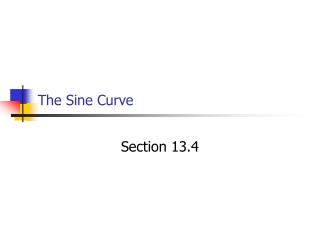DownloadDownload PresentationThe Sine Curve

# The Sine Curve

Télécharger la présentation## The Sine Curve

- - - - - - - - - - - - - - - - - - - - - - - - - - - E N D - - - - - - - - - - - - - - - - - - - - - - - - - - -
##### Presentation Transcript

1. The Sine Curve Section 13.4

2. Objectives… • 1) given an equation for a sine curve, find the period and amplitude • 2) graph a given sine curve equation • 3) given information, write the equation for a sine curve

3. Characteristics of the sine curve (y = sin θ) • graph is periodic (period = 360° or 2 pi radians, amplitude = 1) • corresponds to the y-values of the points where the terminal side of the angle θ intersects the unit circle

4. Illustration of the sine curve (y = sin θ) • http://clem.mscd.edu/~talmanl/HTML/SineCurve.html

5. Drawing/Identifying specific sine curves (y = a sin bθ) • a (absolute value): amplitude (if a < 0, movement of function is the opposite from the normal) • b: # of cycles in the interval from 0 to 2 pi • 2 pi/b: length of the period of the function

6. Drawing specific sine curves… • get all the information needed (period, amplitude, movement of curve) • use the “zero-max-zero-min-zero” method (reverse max and min if a < 0)

7. Examples! • find the period and amplitude of the following sin functions: A) y = sin 6θ B) y = (1/3) sin 4θ C) y = -3 sin 3θ

8. More Examples! • draw the following sin functions from 0 to 2 pi: A) amplitude = 3, period = 4 B) amplitude = 2, period = (3 pi)/4 C) y = 2 sin θ D) y = sin 3θ E) y = 3 sin 4θ F) y = -2.5 sin 2θ G) y = 1.5 sin (1/2)θ H) y = sin (pi/2)θ

9. Writing sine curve equations… • giventhe following periods and amplitudes, write the sine curve equation: A) amplitude = 4; period = (2 pi)/3; a > 0 B) amplitude = 2.5; period = pi; a < 0 C) amplitude = 3; period = (5 pi)/4; a > 0 D) amplitude = 6.25; period = 3; a < 0

10. Homework… • pgs. 724-726, #’s 1-39, 42, 43, 52a,b